# Calculate annuity for Interest rate

For instance, when a series of equal cash flows in equal interval of time is paid. Then we can use the RATE function in excel. But if the intervals at which amounts are paid are not consistent, then use the XIRR function.

The RATE function is used to find the Interest rate of the data set in Excel. It requires the following arguments to calculate the interest rate.

Let’s use it as an example to understand it.
Here we have data having a present value of \$10,000 and amount is paid every month till 60 months (12 years).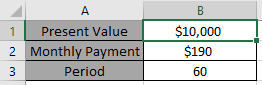We need to find the interest rate on the data provided.

Use the formula

= RATE ( B3 , B2 , - B1 ) * 12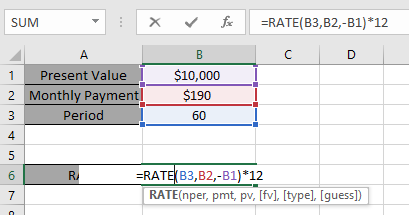Here all the references are given as cell reference as argument to the function.
Press Enter.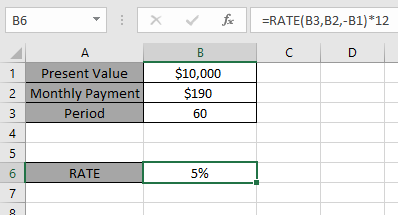The interest rate for the data set is 5%. So it means the interest rate of 5% is paid for the data provided.

Now we will consider one more scenario to calculate annuity for Interest rate.

Here we are given Future value, Present value, annual payment & period of payment is till 7 years.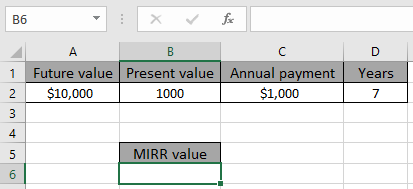We need to find the interest rate on the data provided.
Use the formula

=RATE ( D2 , - C2 , - B2 , A2 )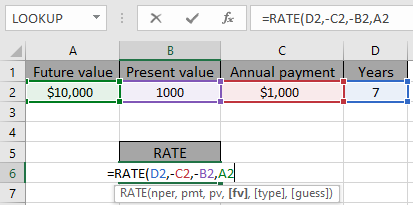Here all the references are given as cell reference as argument to the function. Negative sign is considered with annual payment & present value because credit amount & debit Amount cannot have the same sign.
Press Enter.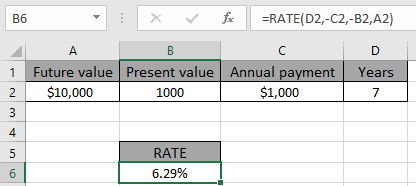The interest rate for the data set is 6.29 %. So it means the interest rate of 6.29 % is paid for the data provided.

Notes:

1. The function provides the #VALUE! error if any argument to the function is non - numeric.
2. The function returns #NUM! error if the credit amount & debit amount signs are not correct. Excel understand negative as the amount received and positive as the amount paid.
3. The RATE function calculates results by iteration and can have zero or more solutions. If the successive results of RATE do not converge to within 0.0000001 after 20 iterations, The function returns the #NUM! error value.

Hope you understand how to use the RATE function to get the Interest rate of the data. Explore more articles on Accounting here.  Please write your queries in the comment box below. We will help you.

Related Articles:

How to Use the RATE Function in Excel

How to Use IRR Function in Excel

How to Use MIRR Function in Excel

How to Use XIRR Function in Excel

Excel IPMT Function

Calculate Profit margin percentage

Excel CUMIPMT Function

Popular Articles:

The VLOOKUP Function in Excel

COUNTIF in Excel 2016

How to Use SUMIF Function in Excel

Terms and Conditions of use

The applications/code on this site are distributed as is and without warranties or liability. In no event shall the owner of the copyrights, or the authors of the applications/code be liable for any loss of profit, any problems or any damage resulting from the use or evaluation of the applications/code.